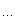# 浪人最终幻想

## 获取HTML表单控件的UrlEncode字符串表达式

/**
*
*  UrlEncode
*  http://www.cnitblog.com/9jian/
*
*
*  调用： UrlEncode.Builder("表单控件id1/表单控件name1",["表单控件idN/表单控件nameN"]);
*  返回： name1=value1&name2=value2&nameN=valueN
*  备注： Ajax应用
*         GET方法   将UrlEncode字符串表达式附加至URL的?字符之后
*
*
*/
var UrlEncode = {
Builder:
function() {

var _urlencode = "", _parameter = "";

for (var i = 0; i < UrlEncode.Builder.arguments.length; i++) {

var ueobj, uename;
uename
= UrlEncode.Builder.arguments[i];
ueobj
= document.getElementsByName(uename);

if (ueobj.length == 0) {
_parameter
= UrlEncode.BuilderParameter(document.getElementById(uename));

if (_parameter.length > 0) {

if (_urlencode.length > 0)
_urlencode
+= "&";
_urlencode
+= _parameter;
}
}

else {

for (var j = 0; j < ueobj.length; j++) {
_parameter
= UrlEncode.BuilderParameter(ueobj[j]);

if (_parameter.length > 0) {

if (_urlencode.length > 0)
_urlencode
+= "&";
_urlencode
+= _parameter;
}
}
}
}

return _urlencode;
},
BuilderParameter:
function(formobj) {

if (formobj == undefined || formobj == null) {

return "";
}

var parameter = "", name = "";
name
= ((formobj.name == undefined || formobj.name == ""? formobj.id : formobj.name);

if (((formobj.type == "checkbox" || formobj.type == "radio"&& formobj.checked) || formobj.type == "text" || formobj.type == "password" || formobj.nodeName == "TEXTAREA") {
parameter
= name + "=" + escape(formobj.value);
}

else if (formobj.nodeName == "SELECT") {

if (formobj.multiple == false) {

if (formobj.value == "")
parameter
= name + "=" + escape(formobj.options[formobj.selectedIndex].text);

else
parameter
= name + "=" + escape(formobj.value);
}

else {

if (formobj.selectedIndex != -1) {

for (var sl = 0; sl < formobj.length; sl++) {

if (formobj.options[sl].selected) {

if (parameter.length > 0)
parameter
+= "&";

if (formobj.options[sl].value == "")
parameter
+= name + "=" + escape(formobj.options[sl].text);

else
parameter
+= name + "=" + escape(formobj.options[sl].value);
}
}
}
}
}

return parameter;
}
}

posted on 2009-04-07 23:33 独孤九剑 阅读(720) 评论(0)  编辑 收藏 引用 所属分类: Web?